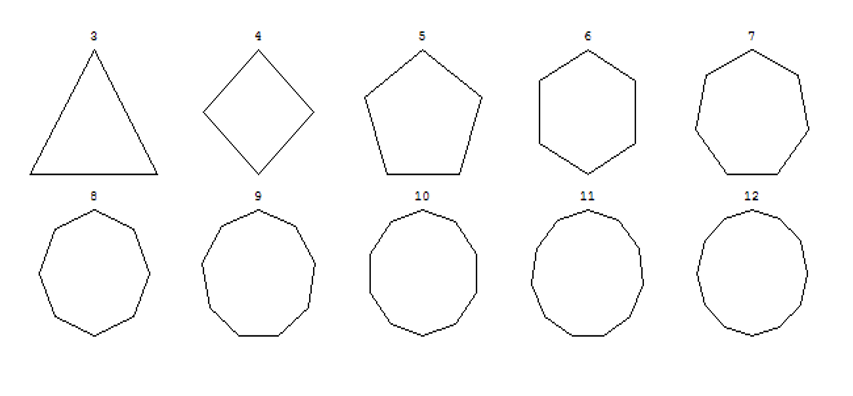# QR2.2.7 The Density of SpaceFigure 2.6 Discrete Rotations, N = 3-12

Space as a network must have a density based on the number of links each node has to others. In the above derivation, this density is the number of steps in each rotation that creates the space. A discrete rotation can have any number of steps, so if a perfect circle has infinite steps, a triangle is a “3-circle”, a square a “4-circle”, a pentagon a “5-circle” and so on (Figure 2.6). These N-circles approximate an ideal circle as N increases. It might seem that more rotation steps is better for a space but war-gamers avoid octagonal nodes because they don’t “fill” a flat board, i.e. octagons placed side-by-side leave gaps. Yet while Euclidean squares fill the board they only give four interaction directions, so war-gamers prefer hexagons as they both fill the board and give more interaction directions.

If the quantum network emulating space is dense, each point will have many connection directions but a large N-circle can’t fill a Euclidean space. So does this exclude it from emulating our space? For example, not all paths in such a space would be reversible, so following a route taken in reverse may not return to the same node, though it would be a true vicinity. In essence, a discrete space based on polar coordinates will have “holes” in it, so billiard ball point particles could pass right through each other!

This might seem to disqualify a space based on discrete rotations for space but objects in our world are better described by quantum clouds than billiard balls. When quantum entities “collide” they overlap over an area, so a space with a few holes in it doesn’t matter. That quantum entities exist inexactly avoids the problems of a polar space. Even so, this model predicts that space as a polar network has a finite number of directions for any quantum event. Quantum realism predicts that direction, like length, is quantized, so there will be a minimum Planck angle.

Next